# OECD Social spending data analysis 6 - Linear regression using RUnsplashWaranont (Joe)が撮影した写真

This post is following of the above post.

In this post I will do linear regression anaysis using R.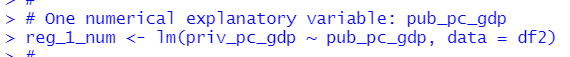Above code shows that explanatory variable id pub_pc_gdp and explained variable is priv_pc_gdp.

Let's see the results with get_regression_table() function and get_regression_summaries() function, the both functions are in moderndive package.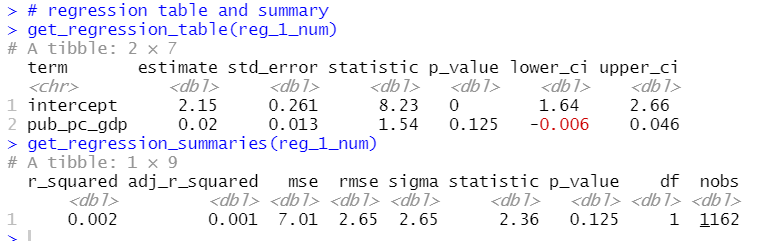pub_pc_gdp's coefficient estimate is 0.02 but lower_ci and upper_ci includes 0, so this implied it is not statistically significant at 95% level.

Next, I use one categorical variable as explanatory variable, it is continent.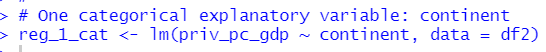Let's see the results.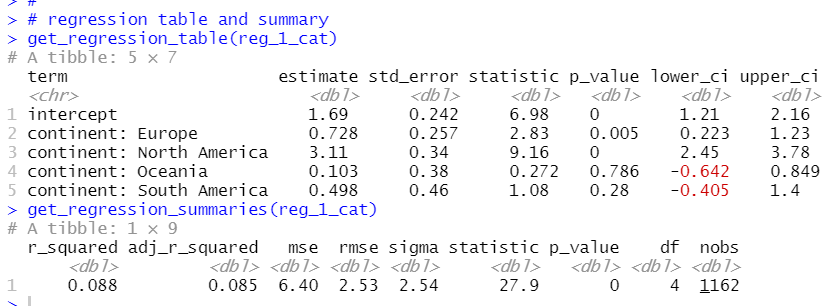Since there is not Asia in the regression table, base continent is Asia. Asia's mean priv_pc_gdp is 1.69 and North America's mean priv_pc_gdp is higer than Asia's mean by 3.11 and it is statistically significant. In addition, Europe's mean is higher than Asia's mean by 0.728 and it is statistically significant.

Next, I use one numerical variable and one categorical variable.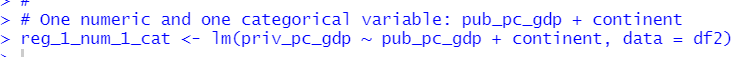Let's see the results.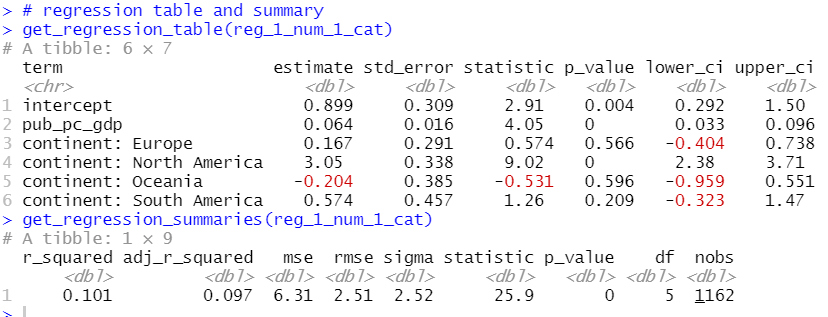When adding continent, pub_pc_gdp becomes statistically significant variable. Europe is no longer statistically significant but North America is still siginificant.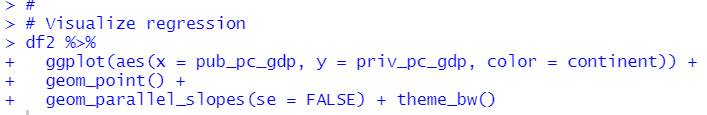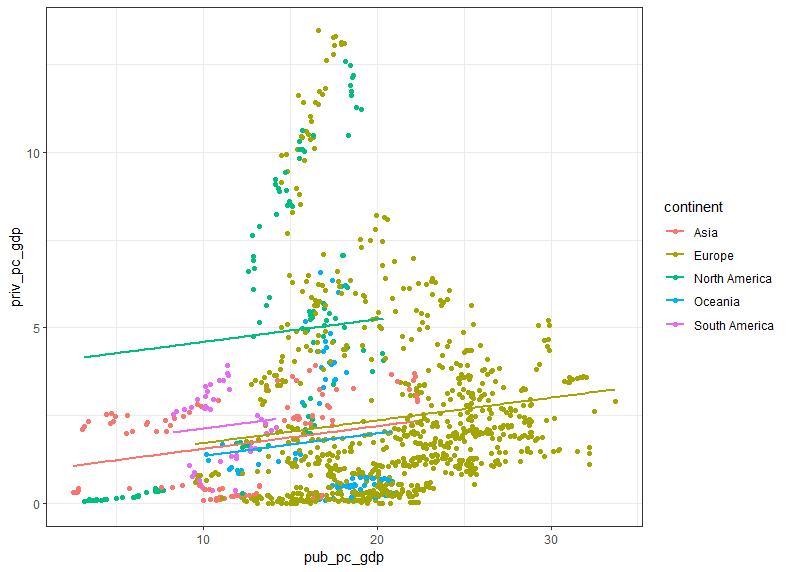moderndive package's geom_parallel_slope() function can add regression line.

Next, I add interaction terms. It means multiple slopes.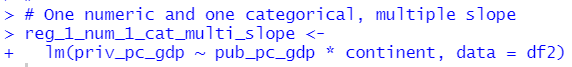pub_pc_gdp * continent makes multiple slopes.

Let's see the results.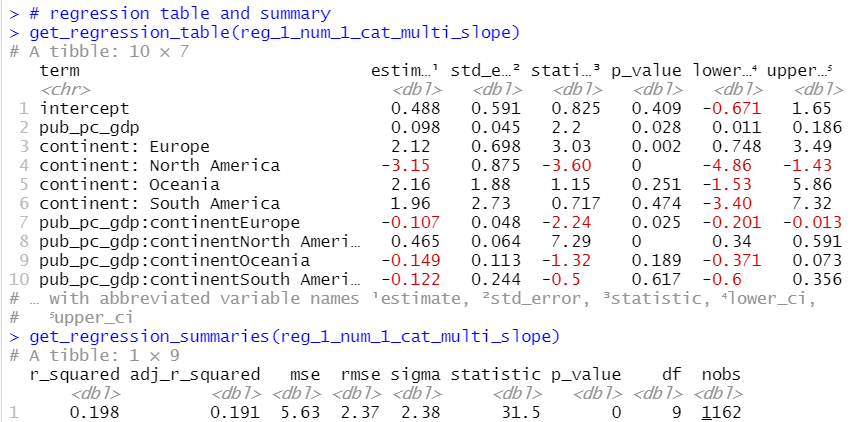Visualize the results.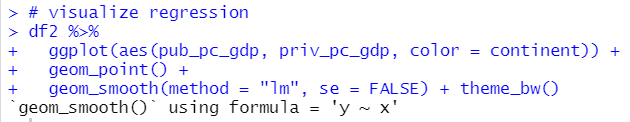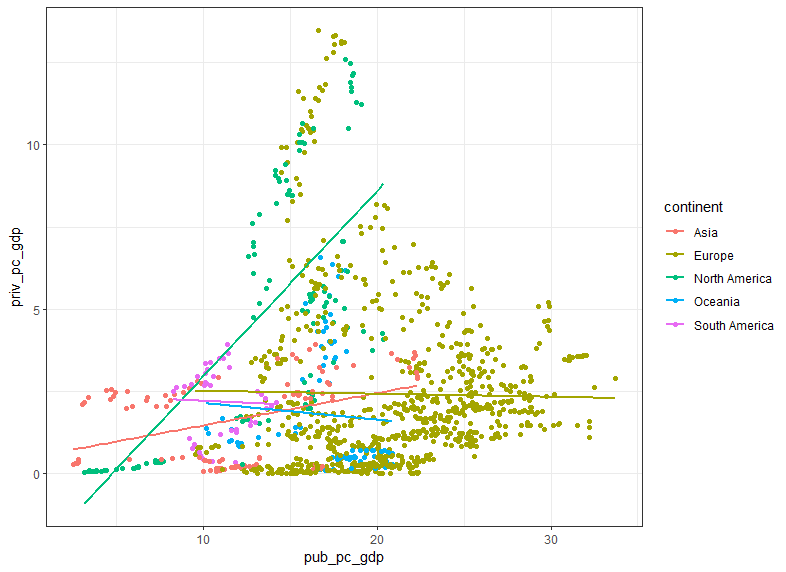I see North Amarica and Asia have positive slopes and Europe, Oceania and South America have negative slopes.

That's it. Thank you!

To read from the first post,